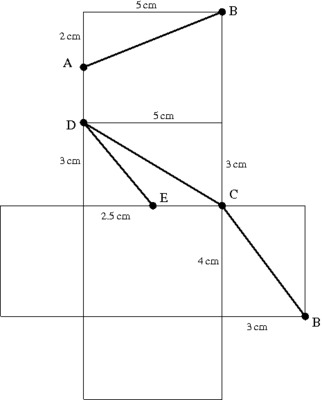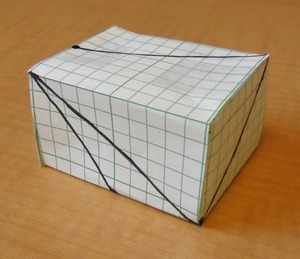# Spiderbox

Alignments to Content Standards: 8.G.B.7

A spider walks on the outside of a box from point A to B to C to D and finally to point E as shown in the picture below.1. Draw a net of the box and map out the path of the spider on the net.
2. Compare your net with those of some other people.
3. Cut out the net and put the box together to see if you are right.
4. How long is the path of the spider?

## IM Commentary

The purpose of this task is for students to work on their visualization skills and to apply the Pythagorean Theorem. The first part of the task actually draws on mathematics students first encounter in 6th grade (see 6.G.4). It is important for students to first try to visualize the two-dimensional path on the surface of the three-dimensional box and then to build it to see if they are correct. It is likely that some students will draw the net with the reflection of the correct path. If the box were built out of a clear material and folded so the path is inside the box, the path would look like it is in the correct place except it would be on the inside. If the box gets folded so the path is on the outside, then it will look like a mirror image of the spider path shown in the figure.

This task allows students to explore how concrete models can help them visualize three-dimensional objects that are drawn in a two dimensional representation. This is part of MP5, Use Appropriate Tools Strategically. Students should have access to plenty of graph paper so they can try again if their first design doesn't work.

## Solution

1.2. Different students may get different nets since the left and right side of the box can be attached to either the top or the bottom or one to each. There are still other possible ways to attach the 6 faces of the box to each other.
3.4. The path consists of 4 line segments. Each line segment is the hypotenuse of a different right triangle.

$\text{length of line segment } AB = \sqrt{2^2+5^2}=\sqrt{29} \approx 5.39\text{ cm}$ $\text{length of line segment } BC = \sqrt{3^2+4^2}=\sqrt{25} =5\text{ cm}$ $\text{length of line segment } CD = \sqrt{3^2+5^2}=\sqrt{34} \approx 5.83\text{ cm}$ $\text{length of line segment } DE = \sqrt{2.5^2+3^2}=\sqrt{15.25} \approx 3.91\text{ cm}$

The total length of the spider's path is about 20.13 cm.